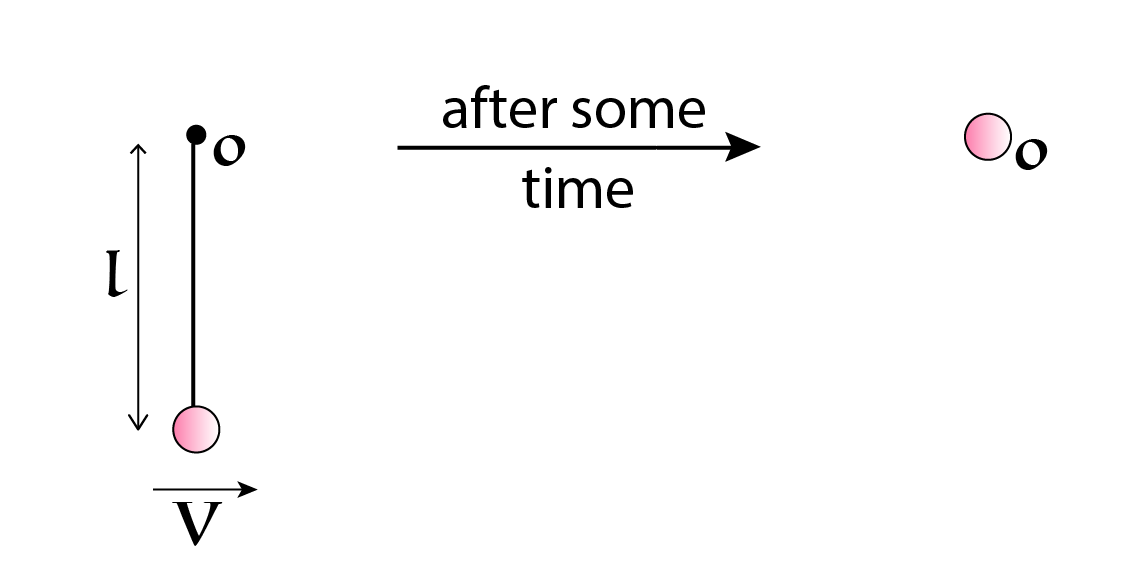# Circular Motion #15A small particle hangs from a fixed point $O$ with the help of a light inextensible string of length $l$.

The particle is initially at rest, at it lowermost point. Now it is given a horizontal velocity $\mathcal { V }$ towards right as shown in the figure above. After some time, the particle passes through $O$.

If the minimum value of $\mathcal{ V }$ satisfying the above condition can be written as $\sqrt{ A g l }$ where $g$ is the gravitational field, find the value of $A$ to $4$ significant figures.

Note: Neglect air resistance.

This problem is part of the set - Circular Motion Practice

×

Problem Loading...

Note Loading...

Set Loading...